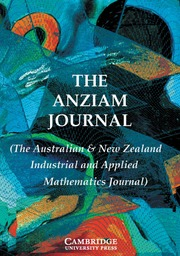Home
Hostname: page-component-7ccbd9845f-mpxzb Total loading time: 0.29 Render date: 2023-01-30T16:12:30.822Z Has data issue: true Feature Flags: { "useRatesEcommerce": false } hasContentIssue trueThe ANZIAM Journal

# The best least squares approximation problem for a 3-parametric exponential regression model

Published online by Cambridge University Press:  17 February 2009

## Abstract

HTML view is not available for this content. However, as you have access to this content, a full PDF is available via the ‘Save PDF’ action button.

Given the data (pi, ti, fi), i = 1,…,m, we consider the existence problem for the best least squares approximation of parameters for the 3-parametric exponential regression model. This problem does not always have a solution. In this paper it is shown that this problem has a solution provided that the data are strongly increasing at the ends.

Type
Research Article
Information
The ANZIAM Journal , October 2000 , pp. 254 - 266

## References

Barrodale, I., Roberts, F. D. K. and Hunt, C. R., “Computing best lp approximations by functions nonlinear in one parameter”, Comput. J. 13 (1970) 382386.CrossRefGoogle Scholar
Demidenko, E. Z., Optimization and regression (in Russian, Nauka, Moscow, 1989).Google Scholar
Golub, G. H. and Pereyra, V., “The differentiation of pseudo-inverses and nonlinear least squares problems whose variables separate”, SIAM J. Numer. Anal. 10 (1973) 413432.CrossRefGoogle Scholar
Jukić, D. and Scitovski, R., “The existence of optimal parameters of the generalized logistic function”, Appl. Math. Comput. 77 (1996) 281294.Google Scholar
Marušić, M. and Bajzer, Ž., “Generalized two-parameter equation of growth”, J. Math. Anal. Appl. 179 (1993) 446462.CrossRefGoogle Scholar
Mitrinović, D. S., Pečarić, J. and Fink, A. M., Classical and New Inequalities in Analysis (Kluwer, Dordrecht, 1993).CrossRefGoogle Scholar
Mühlig, H., “Lösung praktischer Approximationsaufgaben durch Parameteridentifikation”, ZAMM 73 (1993) T837–T839.Google Scholar
Osborne, M. R., “Some special nonlinear least squares problems”, SIAM J. Numer. Anal. 12 (1975) 571592.CrossRefGoogle Scholar
Osborne, M. R. and Smyth, G. K., “A modified Prony algorithm for exponential function fitting”, SIAM J. Sci. Comput. 16 (1995) 119138.CrossRefGoogle Scholar
Ratkowsky, D. A., Handbook of Nonlinear Regression Models (M. Dekker, New York, 1990).Google Scholar
Ross, G. J. S., Nonlinear Estimation (Springer-Verlag, New York, 1990).CrossRefGoogle Scholar
Rudolph, P. E. and Herrendörfer, G., “Optimal experimental design and accuracy of parameter estimation for nonlinear regression models used in long-term selection”, Biom. J. 37 (1995) 183190.CrossRefGoogle Scholar
Ruhe, A. and Wedin, P. A., “Algorithms for separable nonlinear least squares problems”, SIAM Review 22 (1980) 318337.CrossRefGoogle Scholar
Scitovski, R., “A special nonlinear least squares problem”, J. Comput. Appl. Math. 53 (1994) 323331.CrossRefGoogle Scholar
Scitovski, R. and Jukić, D., “A method for solving the parameter identification problem for ordinary differential equations of the second orderAppl. Math. Comput. 74 (1996) 273291.Google Scholar
Scitovski, R. and Jukić, D., “Total least squares problem for exponential function”, Inverse Problems 12 (1996) 341349.CrossRefGoogle Scholar
Späth, H., H, , Numerik (Vieweg, Braunschweig, 1994).CrossRefGoogle Scholar
Varah, J. M., “On fitting exponentials by nonlinear least squares”, SIAM J. Sci. Stat. Comput. 6 (1985) 3044.CrossRefGoogle Scholar
Varah, J. M., “Relative size of the Hessian terms in nonlinear parameter estimation”, SIAM J. Sci. Stat. Comput. 11 (1990) 174179.CrossRefGoogle ScholarYou have Access
7
Cited by

# Save article to Kindle

Note you can select to save to either the @free.kindle.com or @kindle.com variations. ‘@free.kindle.com’ emails are free but can only be saved to your device when it is connected to wi-fi. ‘@kindle.com’ emails can be delivered even when you are not connected to wi-fi, but note that service fees apply.

Find out more about the Kindle Personal Document Service.

The best least squares approximation problem for a 3-parametric exponential regression model
Available formats
×

# Save article to Dropbox

To save this article to your Dropbox account, please select one or more formats and confirm that you agree to abide by our usage policies. If this is the first time you used this feature, you will be asked to authorise Cambridge Core to connect with your Dropbox account. Find out more about saving content to Dropbox.

The best least squares approximation problem for a 3-parametric exponential regression model
Available formats
×

# Save article to Google Drive

To save this article to your Google Drive account, please select one or more formats and confirm that you agree to abide by our usage policies. If this is the first time you used this feature, you will be asked to authorise Cambridge Core to connect with your Google Drive account. Find out more about saving content to Google Drive.

The best least squares approximation problem for a 3-parametric exponential regression model
Available formats
×
×